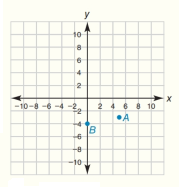Chapter 10.CT, Problem 2CTElementary Geometry For College St...

7th Edition
Alexander + 2 others
ISBN: 9781337614085

Solutions

Chapter
SectionElementary Geometry For College St...

7th Edition
Alexander + 2 others
ISBN: 9781337614085
Textbook Problem

In the coordinate system for Exercise 1, plot and label each point: C ( - 6 ,   1 ) and D ( 0 ,   9 )To determine

To plot:

The given coordinates C(-6, 1) and D(0, 9).

Explanation

Given points are,

C(-6, 1) and D(0, 9)

Point C is located at a distance from origin in the x-axis =-6

Point C is located at a distance from origin in the y-axis =1

Similarly, point D is located at a distance from origin in the x</

Still sussing out bartleby?

Check out a sample textbook solution.

See a sample solution

The Solution to Your Study Problems

Bartleby provides explanations to thousands of textbook problems written by our experts, many with advanced degrees!

Get Started

In Exercises 4562, find the values of x that satisfy the inequality (inequalities). 58. (3x 4)(2x + 2) 0

Applied Calculus for the Managerial, Life, and Social Sciences: A Brief Approach

(y)2 + 6xy 2y + 7x = 0 is a differential equation of order ______. a) 1 b) 2 c) 3 d) 4

Study Guide for Stewart's Single Variable Calculus: Early Transcendentals, 8th

True or False: converges mean exists.

Study Guide for Stewart's Multivariable Calculus, 8th